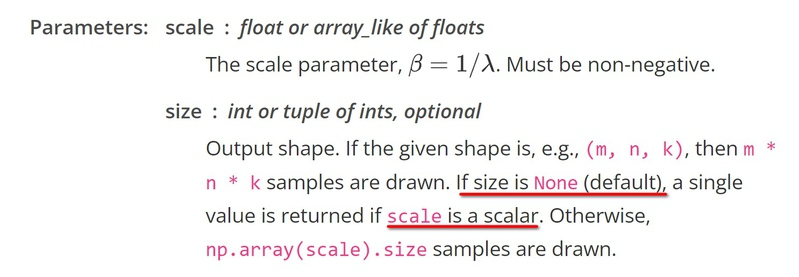Basically we can just use numpy.random.exponential:

```from numpy import random
random.exponential(scale=10, size=None)```

To get scalar from this function we pass size=None according to numpy doc:Let's run this example on 1k elements and see min / max / mean:

```from numpy import random
import statistics
all_nums = [random.exponential(scale=10, size=None) for _ in range(int(10e3))]
print('MIN', min(all_nums))
print('MAX', max(all_nums))
print('AVG', statistics.mean(all_nums))
print('EXAMPLE\n\r', "".join([f"{v:>5.1f}" for i, v in enumerate(all_nums[:30])]))```

We got:

```MIN 0.0029298785466956444
MAX 86.84156021476537
AVG 9.945727975597245
EXAMPLE
17.4  1.5  3.6  4.1  8.7 16.8
20.1  6.0  2.7 33.3  6.5  0.9
1.7  0.6  0.8 21.7  1.9  6.9
2.1 12.0 20.6  0.8  8.9 21.0
11.5  1.1 35.8  1.7 12.5  8.7```

As you can see on scale of 10 on 10k elements we got:

• min: ~0
• max ~86
• so median is 43
• when mean is 9.9

On 100k elements I got:

```MIN 0.0020912108793156
MAX 95.02249624840809
AVG 11.866217176280955```

PS: There is no maximum end because in theory with super-small probability we can get infinity value, but as you can see on 10k draws we able to get value up to 100 on scale 10.

If you need values to start from e.g. 1, just add 1 to the result of function